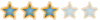Montreal Math ClubMathematics students in Montreal - Étudiants de mathématiques à MontréalHomeCalendarFAQSearchMemberlistUsergroupsRegisterLog in

 Montreal Math Club :: Mathematics :: Problem Solving :: Solved problems Share

# [SOLVED] Primes of the form 8n+1, 8n+3AuthorMessage

AdminPosts : 184
Join date : 2009-09-15
Age : 32
Location : the infinite, frictionless plane of uniform densitySubject: [SOLVED] Primes of the form 8n+1, 8n+3Mon Nov 09, 2009 1:10 am Show that every prime of the form 8n+1 or 8n+3 can be written as a^2+2b^2 for a, b positive integers.(My estimate of the difficulty : 8/10)Last edited by Bruno on Fri Nov 13, 2009 4:32 pm; edited 1 time in totalDescartesPosts : 100
Join date : 2009-11-05
Age : 35
Location : Right behind youSubject: Re: [SOLVED] Primes of the form 8n+1, 8n+3Thu Nov 12, 2009 11:22 pm I am too old to remember some stuffI just know by wonderful Gauss's theorem of quadratic reciprocity it is solvable in mod p. Anyway, I'd love to see the proof and then I will cry why I am far away from my glorious days.AdminPosts : 184
Join date : 2009-09-15
Age : 32
Location : the infinite, frictionless plane of uniform densitySubject: Re: [SOLVED] Primes of the form 8n+1, 8n+3Fri Nov 13, 2009 4:30 pm It is Fermat who conjectured this result.The first step is to show that -2 is a quadratic residue for primes of the form 8n+1 and 8n+3. (In the case of 8n+1, both 2 and -1 are quadratic residues, so -2 is also; in the case of 8n+3, neither 2 nor -1 are quadratic residues, so -2 is.)Thus we can find integers m, k such that m^2+2=kp. Thus (m+√-2)(m-√-2)=kp. Since p divides the left side, but neither of the factors on the right, and since Z[√-2] is a unique factorization domain, p must factor in Z[√-2]. Thus we can write p=(a+b√-2)(c+d√-2). Taking norms on both sides we have p^2=(a^2+2b^2)(c^2+2d^2), so p=a^2+2b^2=c^2+2d^2.(Using the fact that at most two prime ideals in an quadratic integral extension R/Z can lie over a prime ideal in Z, we can also deduce that the representation of p as a^2+2b^2 is unique).DescartesPosts : 100
Join date : 2009-11-05
Age : 35
Location : Right behind youSubject: Re: [SOLVED] Primes of the form 8n+1, 8n+3Fri Nov 13, 2009 8:33 pm oh, I have never studied number theory from this perspective, but there should be another non-machinery proof.AdminPosts : 184
Join date : 2009-09-15
Age : 32
Location : the infinite, frictionless plane of uniform densitySubject: Re: [SOLVED] Primes of the form 8n+1, 8n+3Sat Nov 14, 2009 4:13 am Mohammad wrote:oh, I have never studied number theory from this perspective, but there should be another non-machinery proof.Yes, there is probably another proof!But the algebraic method is elegant.Sponsored contentSubject: Re: [SOLVED] Primes of the form 8n+1, 8n+3[SOLVED] Primes of the form 8n+1, 8n+3Page 1 of 1
 Similar topics» CASH OR MANAGER'S/CASHIER'S CHECK AS THE ONLY FORM OF BID SECURITY
» about ppmp,labor and material
» ITB format
» Anyone used the "lollipop" for severe pain?
» adjacent contiguous contract

Permissions in this forum:You cannot reply to topics in this forumJump to: Select a forum||--Math Club|   |--Mathematical Sundays|   |--Suggestions|   |--Introduce yourself|   |--Mathematics|   |--Problem Solving|   |   |--Solved problems|   |   |   |--Calculus and Analysis|   |--Combinatorics|   |--Geometry|   |--Number Theory|   |--Probabilities and statistics|   |--Set theory and Topology|   |--Online Resources|   |--Chit chat    |--The Water Cooler    |--Mathematical poems